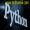# DataFrame添加插入列(赋值法、apply()、assign()、条件筛选、insert())老董2020-06-28160围观,141赞

在DataFrame添加一列常用4种方法，分别是1、直接赋值法; 2、apply函数;3、assign函数; 4、条件筛选，此外，concat函数也可以灵活的添加列，insert函数可以插入列，后续专门讲解这些函数。

1、赋值方法

推荐使用.loc的方式来赋值一列，直接df[xxx]=的方式在一些场景下会有警告，SettingWithCopyWarning: value is trying to be set on a copy of a slice，查看>>SettingWithCopyWarning

```# -*- coding: utf-8 -*-
import pandas as pd

print(df)
print('------------------')

# 数据清洗
df.loc[:,'高温'] = df['高温'].str.replace('度','').astype('int32')
df.loc[:,'低温'] = df['低温'].str.replace('度','').astype('int32')

# 新增列-直接赋值
df.loc[:,'温差'] = df['高温'] - df['低温']
# 新增列-直接赋值
s = pd.Series(['3000万','2000万','1500万','800万'],name='人口')
df.loc[:,'人口'] = s
print(df)
```
```   城市   高温   低温
0  北京  28度  10度
1  上海  25度   7度
2  广州  16度   5度
3  深圳  17度  10度
------------------
城市  高温  低温  温差     人口
0  北京  28  10  18  3000万
1  上海  25   7  18  2000万
2  广州  16   5  11  1500万
3  深圳  17  10   7   800万
```

2、apply函数

```# -*- coding: utf-8 -*-
import pandas as pd

print(df)
print('------------------')

# 数据清洗
df.loc[:,'高温'] = df['高温'].str.replace('度','').astype('int32')
df.loc[:,'低温'] = df['低温'].str.replace('度','').astype('int32')

# axis=1代表对列操作,0则会把'高温'、'低温'当做行索引
df['温差'] = df.apply(lambda x:x['高温'] - x['低温'],axis=1)
print(df)
```
```   城市   高温   低温
0  北京  28度  10度
1  上海  25度   7度
2  广州  16度   5度
3  深圳  17度  10度
------------------
城市  高温  低温  温差
0  北京  28  10  18
1  上海  25   7  18
2  广州  16   5  11
3  深圳  17  10   7
```

3、assign函数

DataFrame.assign(self, **kwargs)，返回一个新的df，可以添加一个或者多个列

```# -*- coding: utf-8 -*-
import pandas as pd

print(df)
print('------------------')

# 数据清洗
df.loc[:,'高温'] = df['高温'].str.replace('度','').astype('int32')
df.loc[:,'低温'] = df['低温'].str.replace('度','').astype('int32')

df2 = df.assign(cha = lambda x:x['高温'] - x['低温'],
sum=lambda x:x['高温'] + x['低温'])
print(df2)
```
```   城市   高温   低温
0  北京  28度  10度
1  上海  25度   7度
2  广州  16度   5度
3  深圳  17度  10度
------------------
城市  高温  低温  cha  sum
0  北京  28  10   18   38
1  上海  25   7   18   32
2  广州  16   5   11   21
3  深圳  17  10    7   27
```

4、条件筛选

条件筛选用法大全可参考：DataFrame的[],loc,iloc多条件判断筛选

```# -*- coding: utf-8 -*-
# -*- coding: utf-8 -*-
import pandas as pd

print(df)
print('------------------')

# 数据清洗
df.loc[:,'高温'] = df['高温'].str.replace('度','').astype('int32')
df.loc[:,'低温'] = df['低温'].str.replace('度','').astype('int32')

df.loc[df['高温'] - df['低温'] >=15,'type'] = '高温差'
df.loc[df['高温'] - df['低温'] <15,'type'] = '低温差'
print(df)
```
```   城市   高温   低温
0  北京  28度  10度
1  上海  25度   7度
2  广州  16度   5度
3  深圳  17度  10度
------------------
城市  高温  低温 type
0  北京  28  10  高温差
1  上海  25   7  高温差
2  广州  16   5  低温差
3  深圳  17  10  低温差
```

6、insert插入1列

```# -*- coding:UTF-8 -*-
import pandas as pd

df = pd.DataFrame({'col1': [1, 2], 'col2': [3, 4]})
col_new = pd.Series(['a','b'])
df.insert(0,'py66',col_new)
print(df)
```
```  py66  col1  col2
0    a     1     3
1    b     2     4
```

python编程网提示：转载请注明来源www.python66.com。

## 文章评论

DataFrame添加插入列(赋值法、apply()、assign()、条件筛选、insert())文章写得不错，值得赞赏

## 站点信息

• 网站程序：Laravel
• 博主微信：a772483200